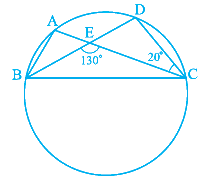# Ex.10.5 Q5 Circles Solution - NCERT Maths Class 9

Go back to  'Ex.10.5'

## Question

In the given figure, $$A, B, C$$ and $$D$$  are four points on a circle. $$AC$$ and $$BD$$  intersect at a point \begin {align}{E} \end {align} such that \begin {align} \angle {BEC}=130^{\circ} \end {align} and \begin {align} \angle {ECD}=20^{\circ}. \end {align} Find \begin {align} \angle {BAC}\end {align}.Video Solution
Circles
Ex 10.5 | Question 5

## Text Solution

What is known?

Two angles  \begin {align} \angle {BEC}=130^{\circ} \end {align}

and \begin {align} \angle {ECD}=20^{\circ} \end {align}

What is unknown?

Value of  $$\angle {BAC}$$

Reasoning:

• Sum of angles in a triangle is $$180^{\circ}.$$
• Angles in the same segment are equal.

Steps:

Consider the straight line \begin {align} {BD} \end {align}. As the line \begin {align} {AC} \end {align} intersects with the line \begin {align} {BD,} \end {align} then the sum of two adjacent angles so formed is $$180^{\circ}.$$

Therefore,

\begin{align} \angle {BEC}+\angle {DEC} &=180^{\circ} \\ 130^{\circ}+\angle {DEC} &=180^{\circ} \\ \angle {DEC} &=180^{\circ}-130^{\circ} \\ &=50^{\circ} \end{align}

Consider the \begin{align} \Delta {DEC,} \end{align} the sum of all angles will be $$180^{\circ}.$$

\begin{align} \angle {DEC}+\angle {EDC}+\angle {ECD} &=180^{\circ} \\ 50^{\circ}+\angle {EDC}+20^{\circ} &=180^{\circ} \end{align}

\begin{align} \angle {EDC} &=180^{\circ}-70^{\circ} \\ &=110^{\circ} \\ ∴ \angle {BDC}=\angle {EDC} &=110^{\circ} \end{align}

We know that, angles in the same segment of a circle are equal.

\begin {align} ∴ \angle {BAC}=\angle {BDC}=110^{\circ}\end {align}

Video Solution
Circles
Ex 10.5 | Question 5

Learn from the best math teachers and top your exams

• Live one on one classroom and doubt clearing
• Practice worksheets in and after class for conceptual clarity
• Personalized curriculum to keep up with school